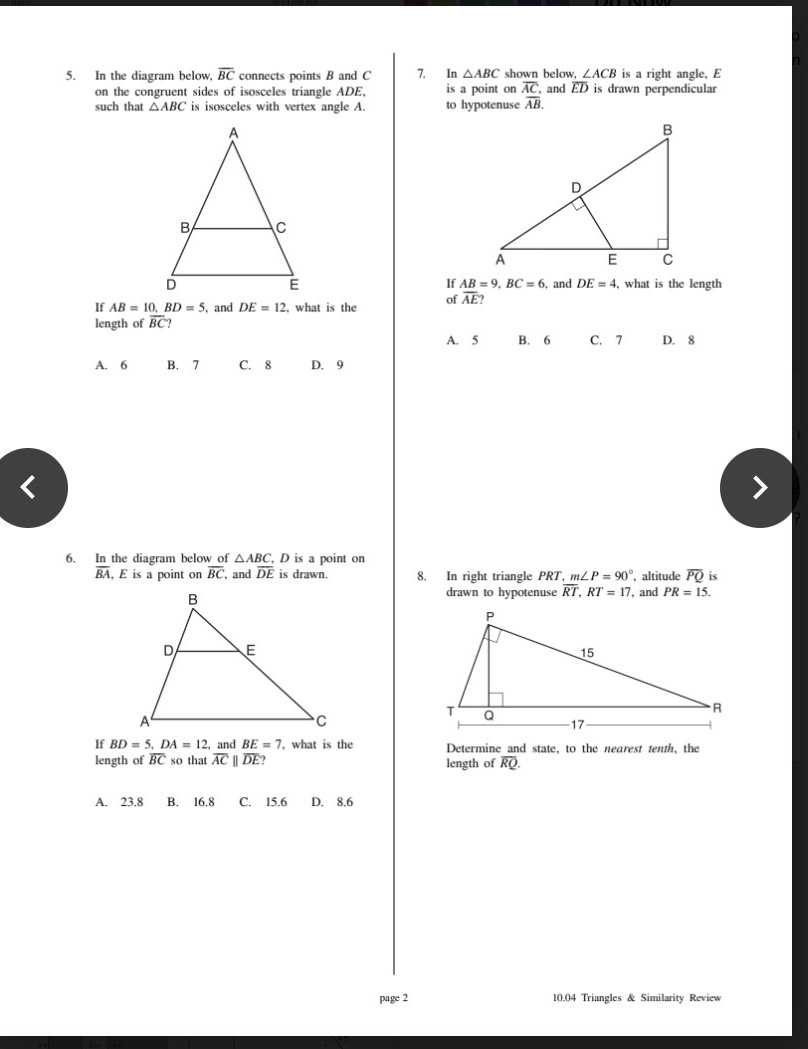### Still have math questions?

Trigonometry
Question5. In the diagram below, $$\overline { B C }$$ connects points $$B$$ and $$C$$ on the congruent sides of an isosceles triangle $$A D E$$ . such that $$\triangle A B C$$ is isosceles with vertex angle $$A$$ .

If $$A B = 10 , B D = 5$$ , and $$D E = 12$$ , what is the length of $$\overline { B C }$$ ?

A. $$6$$               B. $$7$$                     C. $$8$$                   D. $$9$$

6. In the diagram below of $$\triangle A B C , D$$ is a point on $$\overline { B A } , E$$ is a point on $$\overline { B C }$$ , and $$\overline { D E }$$ is drawn.

If $$B D = 5 , D A = 12$$ , and $$B E = 7$$ . what is the length of $$\overline { B C }$$ so that $$\overline { A C } \| \overline { D E }$$ ?

A. $$23.8$$         B. $$16.8$$             C. $$15.6$$            D. $$8.6$$

7. In $$\triangle A B C$$ shown below, $$\angle A C B$$ is a right angle, $$E$$ is a point on $$\overline { A C }$$ , and $$\overline { E D }$$ is drawn perpendicular to hypotenuse $$\overline { A B }$$ .

If $$A B = 9 , B C = 6 ,$$ and $$D E = 4 .$$ what is the length of $$\overline { A E }$$ ?

A. $$5$$                 B. $$6$$                    C. $$7$$                    D. $$8$$

8. In right triangle $$P R T , m \angle P = 90 ^ { \circ }$$ , altitude $$\overline { P Q }$$ is drawn to hypotenuse$$\overline { R T } , R T = 17$$ , and $$P R = 15$$ .

Determine and state, to the nearest tenth, the length of $$\overline { R Q }$$ .